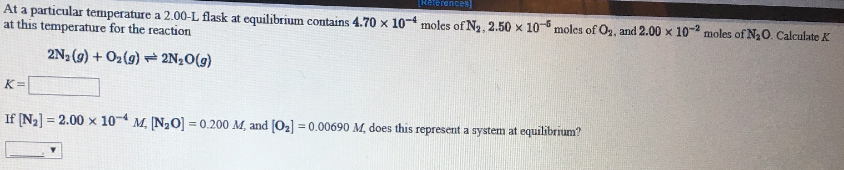# At a particular temperature a 2.00-L flask at equilibrium contains 4.70 x 10^-4 moles of N2, 2.50 x 10^-5 moles of O2, and 2.00 x 10^-2 moles of N2O. Calculate K at this temperature for the reaction 2N2(g) + O2(g) ⇌ 2N2O(g) K = ____ If [N2] = 2.00 x 10^-4 M, [N2O] = 0.200 M, and [O2] = 0.00690 M, does this represent a system at equilibrium?